## solve for y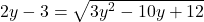absurd answers will be reported!!​

Question

solve for y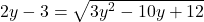in progress 0
2 months 2021-07-30T08:09:56+00:00 2 Answers 2 views 0

y = 3

Step-by-step explanation:

2y – 3 =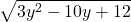square both sides to remove sqrt bracket

(2y – 3)^2 = ()^2

simplify both sides

(2y – 3)(2y – 3) =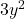– 10y + 12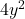– 12y + 9 =– 10y + 12

bring all value to left side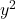– 2y – 3 = 0

factor

(y – 3)(y + 1)

solve for y

y = 3, y = -1

When plugged back into the equation, only y = 3 is true

y = 3

Step-by-step explanation :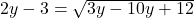Swap the sides both of the equation.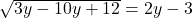To remove the brackets of equations square both side and simplify .

3y² – 10y + 12 = 4y² – 12y + 9

Move the expression to left-hand side and change its sign.

3y² – 10y + 12 – 4y² + 12y – 9 = 0

collect like terms

3y² – 4y² – 10y + 12y + 12 – 9 = 0

-y² + 2y + 3 = 0

Change the sign of expression. because it helps to solve.

y² – 2y – 3 = 0

Splits the term -2y

y² + y 3y – 3 = 0

Factor out y from the first pair and -3 from second pair of expression.

y ( y + 1 ) – 3 ( y + 1) = 0

Factor out y + 1 from the expression.

( y + 1 ) ( y – 3 ). = 0

When product and factors equals 0. at least one factor is 0.

y + 1 =0

y – 3 = 0

Solve for y

y = -1 and y = 3

If we plug the 3 as y in the expression we find that y = 3 is the true solution of this expression.

This equation has one solution which is y = 3.